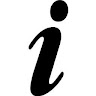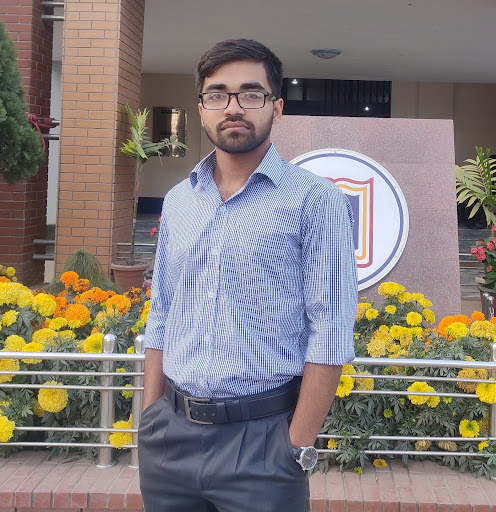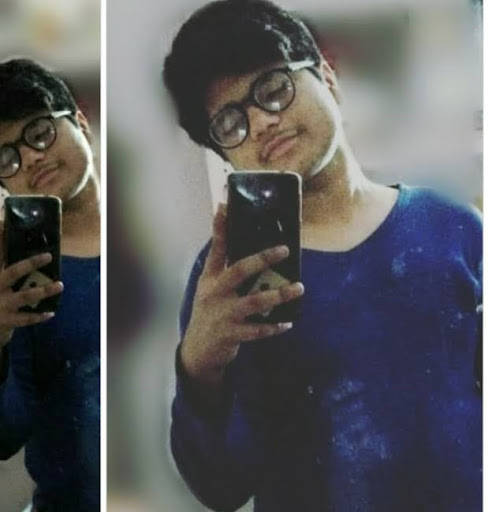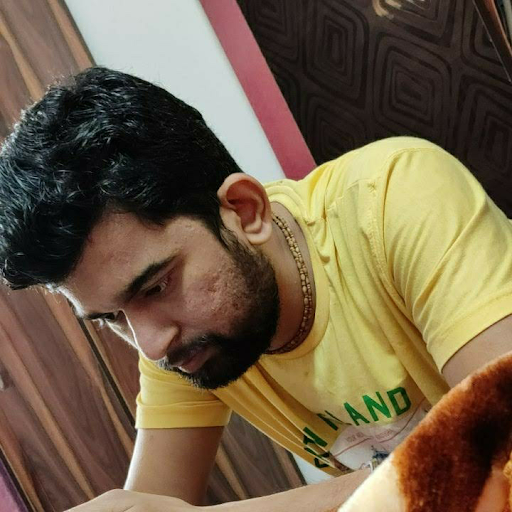# How many different words can be formed using all the letters of the word ALLAHABAD? (a) When vowels occupy the even positions. (b) Both L do not occur together.

A. 7560,60,1680

B. 7890,120,650

C. 7650,200,4444

D. None of these

### Solution(By Examveda Team)

ALLAHABAD = 9 letters. Out of these 9 letters there is 4 A's and 2 L's are there.
So, permutations = $$\frac{{9!}}{{4!.2!}}$$ = 7560

(a) There are 4 vowels and all are alike i.e. 4A's.
_2nd _4th _6th _8th _
These even places can be occupied by 4 vowels. In $$\frac{{4!}}{{4!}}$$ = 1 Way.
In other five places 5 other letter can be occupied of which two are alike i.e. 2L's.
Number of ways = $$\frac{{5!}}{{2!}}$$ Ways.
Hence, total number of ways in which vowels occupy the even places = $$\frac{{5!}}{{2!}}$$ × 1 = 60 ways.

(b) Taking both L's together and treating them as one letter we have 8 letters out of which A repeats 4 times and others are distinct.
These 8 letters can be arranged in $$\frac{{8!}}{{4!}}$$ = 1680 ways.
Also two L can be arranged themselves in 2! ways.
So, Total no. of ways in which L are together = 1680 × 2 = 3360 ways.
Now,
Total arrangement in which L never occur together,
= Total arrangement - Total no. of ways in which L occur together.
= 7560 - 3360
= 4200 ways

1.Also two L can be arranged themselves in 2!/2!=1 ways.
Total arrangement in which L never occur together,
= Total arrangement - Total no. of ways in which L occur together.
= 7560 - 1680
= 5880 ways

2.I had confusion in the last part.
2 L can't be arranged in 2 way. It can be arranged in one way.
Thanks folks

3.2 nd part 5880

4.5.[9!/(4!2!)] - [8!2!/4!2!]
=7560-1680
=5880

6.7.HI,
thanks for this wonderful stuff. I have a concern with last part of this question where you multiplied 1680 by saying both L can be arranged in two ways by themselves. Is it correct if both is the same word?

Kindly reply if you really have started this forum for the visitors, not for the sake of "Link Building for search engines"

Best Regards

8.answer should be none of these and for part b it is 5880 ways

9.Answer given for option B should be as given by Sajna Salas and Sonveer Tomar

10.Please correct the answer of option B as explained in above comment

11.The second part of the question is asked as
b) Both L do not occur together

But the solution given is for
(b) Taking both L's together and treating them as one letter we have 8 letters out of which A repeats 4 times and others are distinct.
These 8 letters can be arranged in 81/4! = 1680 ways.

So I think the answer should be Total no. of arrangements in which vowels are never together:Total no. of arrangements in which vowels are never together:

Total no. of arrangements in which L's are never together:

=ALL the arrangements possible - arrangements in which L's are ALWAYS TOGETHER
= 7560-1680 = 5880 ways .

Related Questions on Permutation and Combination